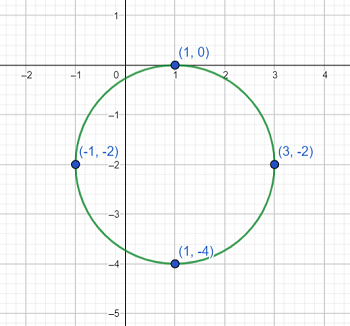# Use the following parametric equations x = 1 + 2cost 0 \leq t \leq 2\pi x = - 2 + 2sint (a) Graph...

## Question:

Use the following parametric equations

x = 1 + 2cost {eq}0 \leq t \leq 2\pi {/eq}

x = - 2 + 2sint

(a) Graph the parametric equations. Clearly label four points on the graph with the coordinates, (x, y), and the corresponding parametric values, 't'.

(b) Determine the rectangular or Cartesian equation for the system by eliminating the parameter.

## Parametric Equations:

To graph a parametric equation, we are often reduced to making a table to get some representative points, and then playing connect-the-dots. Instead, we can convert the equation immediately and use our familiarity with equations in Cartesian coordinates to plot the curve.

Part B

Since we need to get the Cartesian equation anyway, we may as well take advantage of it to plot the curve (this problem is a great example of how to do things backwards; we will be doing the parts in a much more natural order). Since we have a cosine and sine function, we take advantage of the Pythagorean identity {eq}\cos^2 t + \sin^2 t = 1 {/eq}. Note that we can write

{eq}\begin{align*} x &= 1 + 2 \cos t \\ x-1 &= 2 \cos t \\ \cos t &= \frac{x-1}2 \end{align*} {/eq}

and

{eq}\begin{align*} y &= -2 + 2\sin t \\ y+2 &= 2\sin t \\ \sin t &= \frac{y+2}2 \end{align*} {/eq}

So the curve is really

{eq}\begin{align*} \cos^2 t + \sin^2 t &= 1 \\ \left( \frac{x-1}2 \right)^2 + \left( \frac{y+2}2 \right)^2 &= 1 \\ (x-1)^2 + (y+2)^2 &= 2^2 \end{align*} {/eq}

which is just a circle with radius 2 centered at {eq}(1,-2) {/eq}. Note that the limits on {eq}t {/eq} get us once around the circle.

Part A

Now that we know exactly what the curve is, finding points is trivially simple. We plot the circle, and plot its four extreme points, ending up withNote that starting at the rightmost point, and going around counterclockwise, we are at {eq}t = 0,\ \frac\pi2,\ \pi,\ \frac{3\pi}2 {/eq} respectively.